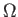Next: Graph Connectivity Up: Graph Properties Previous: Representing Graphs as Bit   Contents

# Non-trivial Monotone Graph Properties

For a non-trivial monotone graph property p, there exists some a < b such that the property holds for all graphs with b edges, but not for any graph with a edges. We can therefore apply Theorem 2.5.1. While this may yield a good lower bound, sometimes it will give us a trivial(1) lower bound.

SubsectionsNext: Graph Connectivity Up: Graph Properties Previous: Representing Graphs as Bit   Contents
Matthew Hayward Lower Query Bounds in the Quantum Oracle Model GitHub Repository